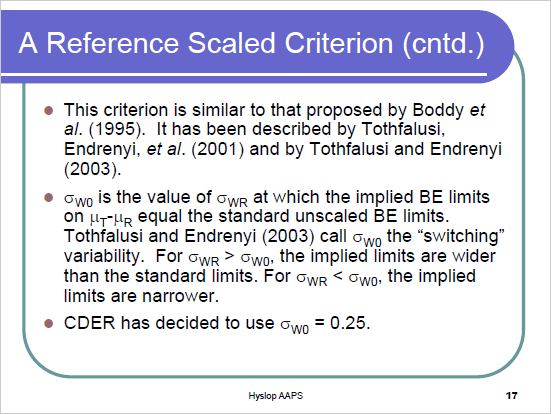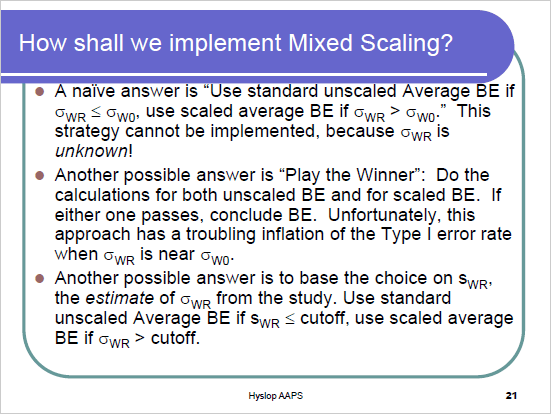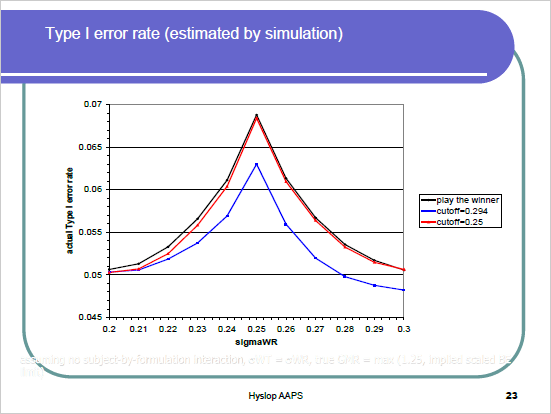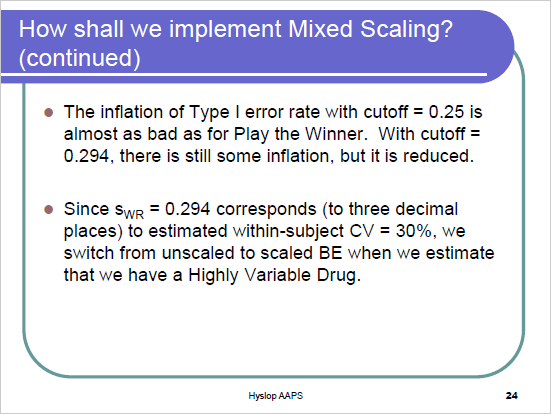Mikalai
☆

Belarus,
2019-11-05 18:59

Posting: # 20750
Views: 348

## Inflation type one error [RSABE / ABEL]

Dear all,

1. I am not a statistician and struggle to grasp the concept of type one error inflation in the reference-scaled approach. We basically do the same things as in the usual average bioequivalence where we are able to preserve TIE at 5%, but when we expand CI, we have got TIE inflation. What is behind this inflation, philosophically and mathematically? I also bumped into this discussion where some ever argue about whether this concept even exists. https://daniellakens.blogspot.com/2016/12/why-type-1-errors-are-more-important.html I assume this is not related to multiple testing. Also, we have a statistical concept, but do we have any real proof of this concept? Does anyone know products that were initially registered and then withdrawn from the market because their initial bioequivalence had been due to the inflation TIE.
2 Maybe this is not related to SABE but two-stage design, but anyway. What prevents us from using Bonferroni correction in the two-stage adaptive design, instead of rather complicated other statistical approaches?

Helmut
★★★Vienna, Austria,
2019-11-05 22:50

@ Mikalai
Posting: # 20751
Views: 321

## Inflation type one error

Hi Mikalai,

» I am not a statistician […]

So am I.» We basically do the same things as in the usual average bioequivalence where we are able to preserve TIE at 5%, [... ]

No, we aren’t. In ABE we have fixed limits of the acceptance range, i.e. a pre-specified Null Hypothesis. In ABEL the limits are random variables or in other words, the Null is generated ‘in face of the data’. That means that each study sets it own standards and if we have a couple of HVDPs, each of them was approved according to different rules.

» What is behind this inflation, philosophically and mathematically?

Maybe this presentation helps. In short: Reference-scaling is based on the true population parameters (hence the Greek letters $\theta_s,\,\mu_T,\,\mu_R,\,\sigma_{wR}$). The true standard deviation $\sigma_{wR}$ of the reference is unknown. We have only its estimate $s_{wR}$ from the study. Imagine: The true within-subject CV of the reference is 27%. Hence, it is not an HVD(P) and we should use the conventional limits of 80.00-125.00%. However, by chance in our study we get an estimate of 35% and we expand the limits. Since the PE and the 90% are not affected it means that the chance of passing BE increases. The chance to falsely not accepting the Null increases and this is the inflated type I error.

» I also bumped into this discussion where some ever argue about whether this concept even exists. https://daniellakens.blogspot.com/2016/12/why-type-1-errors-are-more-important.html I assume this is not related to multiple testing.

Nice one. Your assumption is correct.

» Also we have a statistical concept, but do we have any real proof of this concept? Does any» W know products that were initially registered and then withdrawn from the market because their initial bioequivalence had been due to the inflation TIE?

No (twice). But these questions diserve a detailed discussion. More when I’ll be back from Athens.

Cheers,
Helmut SchützThe quality of responses received is directly proportional to the quality of the question asked. ☼
Science Quotes
Mikalai
☆

Belarus,
2019-11-06 15:13

@ Helmut
Posting: # 20756
Views: 254

## Inflation type one error

» Maybe this presentation helps. In short: Reference-scaling is based on the true population parameters (hence the Greek letters $\theta_s,\,\mu_T,\,\mu_R,\,\sigma_{wR}$). The true standard deviation $\sigma_{wR}$ of the reference is unknown. We have only its estimate $s_{wR}$ from the study. Imagine: The true within-subject CV of the reference is 27%. Hence, it is not an HVD(P) and we should use the conventional limits of 80.00-125.00%. However, by chance in our study we get an estimate of 35% and we expand the limits. Since the PE and the 90% are not affected it means that the chance of passing BE increases. The chance to falsely not accepting the Null increases and this is the inflated type I error.

Dear Helmut,
I may be wrong but I cannot see how we can get a true within-subject CV of any drug. I may be wrong, but even with simulations (I suppose with simulations some assumptions regarding variance should be made), it is very difficult or even impossible. Usually, we have very scarce data on within-subject CVs. How can we control in this situation TIE and what regulators say on this subject? I do not remember any reflection on this matter in official documents (EMA, FDA)?
Regards,
Mikalai
Helmut
★★★Vienna, Austria,
2019-11-08 14:52

@ Mikalai
Posting: # 20766
Views: 194

## Inflation type one error

Hi Mikalai,

» I may be wrong but I cannot see how we can get a true within-subject CV of any drug.

Of course, you are right. The true CVwR is unknown. But reference-scaling should be done for true HVD(P)s, i.e., where the population’s CVwR >30%. However, $s_{wR}$ is the best unbiased estimate of $\sigma_{wR}$. The former is used in the expansion formula, i.e., treating $s_{wR}$ as a true value.

» I may be wrong, but even with simulations (I suppose with simulations some assumptions regarding variance should be made), it is very difficult or even impossible.

There are essentially two options:
1. Our ad hoc solution1 by simulating under the assumption $s_{wR}=\sigma_{wR}$ to iteratively adjust $\alpha$. That’s in the “spirit of the guideline” where the observed CVwR is used for expanding the limits.
2. Muñoz et al.2 suggested to “assume the worst” and – since the true value is unknown – adjust $\alpha$ always as if CVwR = 30%. That’s in any case the most conservative approach but might negatively impact power in case of high CVs (were the upper cap of scaling and the GMR-restriction already effectively controls the TIE). For examples see there.
Note that in both approaches the GMR of the Null is specified according to the expanded limits.

Still: The expansion is based on the observed CVwR. We once had the crazy idea of using a very conservative (99.9%) CI instead. Doesn’t work because then we would practically never be allowed to scale…

» […] what regulators say on this subject?

Nothing. I raised this issue at numerous conferences. Dead silence. Armin Koch (co-author of one of the papers3 noting the inflated TIE) is a member of the EMA’s Biostatistical Working Party. Sent him an e-mail in 2016. No answer.» I do not remember any reflection on this matter in official documents (EMA, FDA)?

EMA = zero. At the 2nd GBHI conference (Sep 2016, Rockville) László Endrenyi gave a presentation “Features, Constraints, and Extensions of the Scaling Approach” where he showed examples of the TIE, both for the EMA’s and the FDA’s approaches. Donald Schuirmann said “There is a recent paper in Pharm Res. showing how to deal with the inflation of the type I error. This is an excellent and applicable approach.” and told me in a coffee-break “… if this is correct, we have to modify our method”. Didn’t happen. Will ask him again next month at the 4th GBHI in Bethesda.

1. Labes D, Schütz H. Inflation of Type I Error in the Evaluation of Scaled Average Bioequivalence, and a Method for its Control. Pharm Res. 2016: 33(11); 2805–14. doi:10.1007/s11095-016-2006-1.
2. Muñoz J, Alcaide D, Ocaña J. Consumer’s risk in the EMA and FDA regulatory approaches for bioequivalence in highly variable drugs. Stat Med. 2016: 35(12); 1933–43. doi:10.1002/sim.6834.
3. Wonnemann M, Frömke C, Koch A. Inflation of the Type I Error: Investigations on Regulatory Recommendations for Bioequivalence of Highly Variable Drugs. Pharm Res. 2015: 32(1); 135–43. doi:10.1007/s11095-014-1450-z.

Cheers,
Helmut SchützThe quality of responses received is directly proportional to the quality of the question asked. ☼
Science Quotes
Helmut
★★★Vienna, Austria,
2019-11-10 11:33

@ Mikalai
Posting: # 20780
Views: 84

## Inflation type one error: FDA

Hi Mikalai,

» […] what regulators say on this subject? I do not remember any reflection on this matter in official documents (EMA, FDA)?

Some slides of Terry Hyslop (Director, Division of Biostatistics) of his presentation “Bioequivalence (BE) for Highly Variable Drugs” at the AAPS Workshop (New Orleans, Nov 2010) – after the progesterone guidance was published…Aka the ‘implied limits’ (see this post and slide 23 below).Well roared, lion! Trouble starts because we use $s_{WR}$ instead of the unknown $\sigma_{WR}$.
Nasty but $s_{WR}$ is all we have.
… use scaled average BE if sWR > cutoff.Illegible text (white with grey shadowing):
assuming no subject-by-formulation interaction, σWT = σWR,
true GMR = max (1.25, implied scaled BE limit)Check:

library(PowerTOST) res        <- data.frame(method = c("ABE", "RSABE"), TIE = NA) res$TIE <- power.TOST(CV = 0.3, n = 36, theta0 = 1.25, design ="2x3x3") res$TIE <- power.RSABE(CV = 0.3, n = 36, theta0 = 1.25,                           design ="2x3x3", nsims = 1e6) res$TIE <- signif(res$TIE, 4) print(res, row.names = FALSE) # method    TIE #    ABE 0.0500 #  RSABE 0.1323

Hence, the FDA was well aware of the inflated type I error and decided to ignore it.

Cheers,
Helmut SchützThe quality of responses received is directly proportional to the quality of the question asked. ☼
Science Quotes
PharmCat
☆

Russia,
2019-11-05 23:06

@ Mikalai
Posting: # 20752
Views: 310

## Inflation type one error

» Dear all,

Dear Mikalai, Bonferroni correction performed when two o more independent tests done. And this correction is very rude (More delicate is Sidak correction). But in case of adaptive design we have one test, and then another with part of same data. We don't have independent comparation and we should spend our alpha: one part in first test, another at second. And we can spend any proportion of alpha as we wish, but overal alpha should not be greater, for example 0.05. We should use an application of alpha-spending function. Pocock boundary, Haybittle–Peto boundary, O'Brien–Fleming boundary - there are many approaches to work with interim analysis.

Range of CI itself don't influence on TIE, it is only convention. But when CI dynamically changing I think there is no good definition for TIE. For fixed CI TIE means that real GMR may be outside permissible range with this chance. Very touching assumption to consider that TIE for RSABE is a chance when GMR outside 0.8-1.25 and with this comprehension make CI range wider. Really in this situation TIE not the same as in fixed case. But people want to make ABE for high-variable drug and try to do thisit's like attempt to trick statistics...

But I could be wrong...
Helmut
★★★Vienna, Austria,
2019-11-08 15:21

@ PharmCat
Posting: # 20767
Views: 190

## Inflation type one error

Hi PharmCat,

» But when CI dynamically changing I think there is no good definition for TIE.

I guess you mean that the acceptance range changes (depending on the $s_{wR}$). The CI is not affected.

» For fixed CI TIE means that real GMR may be outside permissible range with this chance.

Yep. For fixed limits the TIE is defined based on the Null of bioinquivalence. Directly accessible as the power for GMR exactly at one of the limits.

library(PowerTOST) CV     <- 0.3 n      <- 34 design <- "2x2x4" GMR    <- 1.25 # exact power.TOST(CV = CV, n = n, theta0 = GMR, design = design) #  0.05 # simulations power.TOST.sim(CV = CV, n = n, theta0 = GMR, design = design, nsims = 1e6) #  0.050097

You can plug in any CV, n, design and the TIE will never exceed nominal α.

» Very touching assumption to consider that TIE for RSABE is a chance when GMR outside 0.8-1.25 and with this comprehension make CI range wider. Really in this situation TIE not the same as in fixed case.

Here the trouble starts (see what I wrote above). What we are doing here is actually HARKing (Hypothesizing After the Results are Known). Not exactly but we definitely generate the Null from the data. Apart from the TIE-issues every product approved by RSABE/ABEL followed its own rules. From a consumer’s perspective this is not fortunate.

» But people want to make ABE for high-variable drug and try to do thisit's like attempt to trick statistics...

Not sure what you mean here. Can you elaborate?

Cheers,
Helmut SchützThe quality of responses received is directly proportional to the quality of the question asked. ☼
Science Quotes
PharmCat
☆

Russia,
2019-11-08 18:15

@ Helmut
Posting: # 20769
Views: 181

## Inflation type one error

Hello!

» Here the trouble starts (see what I wrote above). What we are doing here is actually HARKing (Hypothesizing After the Results are Known). Not exactly but we definitely generate the Null from the data. Apart from the TIE-issues every product approved by RSABE/ABEL followed its own rules. From a consumer’s perspective this is not fortunate.

Yes, we generate hypothesis, but we loss any link of TIE with reality. We form hypothesis from variance estimate, but it is only estimate we don't know real variance. I can't imagine how to definite TIE in this case.

» Not sure what you mean here. Can you elaborate?

Ofcource. It it my description of situationI think that "HARKing" come to bioequivalce because it is very expencive to make BE with big samplesize or make theraputic equivalence and it is compromise between regulators and industry. And from my side HARKing is a bad statistics (yes: consumer’s perspective this is not fortunate), but it is discussible ... some persons recommending that HARKing not be taught by educators, encouraged by reviewers or editors, or practiced by authors
Helmut
★★★Vienna, Austria,
2019-11-08 20:26

@ PharmCat
Posting: # 20770
Views: 162

## TIE = chance of passing at the border(s)

Hi PharmCat,

» Sorry for my bad english!

No worries, mine is hardly better.

» Yes, we generate hypothesis, but we loss any link of TIE with reality. We form hypothesis from variance estimate, but it is only estimate we don't know real variance.

Well, the expansion according to the guideline(s) uses the estimate as well. Try this one:

library(PowerTOST) theta0   <- seq(0.75, 1, 0.01) theta0   <- sort(unique(c(theta0, 1/theta0))) CV       <- 0.30 design   <- "2x2x4" n        <- sampleN.scABEL(CV = CV, design = design, theta0 = 0.90,                            print = FALSE, details = FALSE)[["Sample size"]] powerRSABE.ad <- powerRSABE <- powerABEL.ad <- powerABEL <- powerABE <- numeric() ABEL.ad  <- scABEL.ad(CV = CV, n = n, design = design, print = FALSE)$alpha.adj RSABE.ad <- scABEL.ad(CV = CV, n = n, design = design, regulator = "FDA", print = FALSE)$alpha.adj for (j in seq_along(theta0)) {   if (theta0[j] == 0.80 | theta0[j] == 1.25) nsims <- 1e6 else nsims <- 1e5   powerABE[j]      <- power.TOST(CV = CV, theta0 = theta0[j], n = n,                                  design = design)   powerABEL[j]     <- power.scABEL(CV = CV, theta0 = theta0[j], n = n,                                    design = design, nsims = nsims)   powerABEL.ad[j]  <- power.scABEL(alpha = ABEL.ad, CV = CV, theta0 = theta0[j],                                    n = n, design = design, nsims = nsims)   powerRSABE[j]    <- power.RSABE(CV = CV, theta0 = theta0[j], n = n,                                   design = design, nsims = nsims)   powerRSABE.ad[j] <- power.RSABE(alpha = RSABE.ad, CV = CV, theta0 = theta0[j],                                   n = n, design = design, nsims = nsims) } plot(theta0, powerABE, type = "n", log = "x", lwd = 2, las = 1,      ylab = "chance of passing") grid() col <- c("#00AA00", "red", "blue", "magenta", "grey25") abline(v = c(0.80, 1.25), col = "grey75") abline(h = 0.05, lty = 2, col = "red") lines(theta0, powerABE, lwd = 2, col = col) lines(theta0, powerABEL, lwd = 2, col = col) lines(theta0, powerABEL.adj, lwd = 2, col = col) lines(theta0, powerRSABE, lwd = 2, col = col) lines(theta0, powerRSABE.ad, lwd = 2, col = col) legend("center", bg = "white", box.lty = 0, text.col = col,        legend = c("ABE", "ABEL (\u03B1 0.05)",                   paste0("ABEL (\u03B1 ", signif(ABEL.adj, 3), ")"),                   "RSABE (\u03B1 0.05)",                   paste0("RSABE (\u03B1 ", signif(RSABE.adj, 3), ")"))) powerABE[which(theta0 == 0.80 | theta0 == 1.25)] #  0.05 0.05 powerABEL[which(theta0 == 0.80 | theta0 == 1.25)] #  0.081285 0.081626 powerABEL.ad[which(theta0 == 0.80 | theta0 == 1.25)] #  0.049751 0.050000

With a true CV of 30% we are not allowed to scale but the chance of passing with ABEL is higher than with ABE.
In ~50% of studies we will observe a CV of >30% ($s_{wR} >0.294$) and expand the limits although the drug is not highly variable in the population ($\sigma_{wR} \leq0.294$). The fact that more than 5% pass at each of the borders of the acceptance range is a nasty side effect.

» I can't imagine how to definite TIE in this case.

In analogy to ABE (where the TIE is the chance of passing at the borders of the acceptance range) all authors (with one exception1) of papers dealing with RSABE/ABEL employed the borders of expanded limits. IMHO, that’s a natural choice.
Davit et al.1 distinguished between the ‘implied limits’ and the limits of the ‘desired consumer risk model’. The FDA assessed the TIE at the border of the latter, which decreases the TIE. I believe it that the FDA desires something but in actual studies one has to follow the guidance ending up with the former…

res <- data.frame(CV = sort(c(seq(0.25, 0.32, 0.01), se2CV(0.25))),                   impl.L = NA, impl.U = NA, impl.TIE = NA,                   des.L = 0.80, des.U = 1.25, des.TIE = NA) for (j in 1:nrow(res)) {   res[j, 2:3] <- scABEL(CV = res$CV[j], regulator = "FDA") if (CV2se(res$CV[j]) > 0.25) { # Hey presto, hocus-pocus!     res[j, 5:6] <- exp(c(-1, +1)*(log(1.25)/0.25)*CV2se(res$CV[j])) } res[j, 4] <- power.RSABE(CV = res$CV[j], theta0 = res[j, 3],                            design = "2x2x4", n = 32, nsims = 1e6)   res[j, 7] <- power.RSABE(CV = res\$CV[j], theta0 = res[j, 5],                            design = "2x2x4", n = 32, nsims = 1e6) } print(signif(res, 4), row.names = FALSE) #    CV impl.L impl.U impl.TIE  des.L des.U des.TIE # 0.250 0.8000  1.250  0.06068 0.8000 1.250 0.06068 # 0.254 0.8000  1.250  0.06396 0.8000 1.250 0.06396 # 0.260 0.8000  1.250  0.07008 0.7959 1.256 0.05731 # 0.270 0.8000  1.250  0.08352 0.7892 1.267 0.05098 # 0.280 0.8000  1.250  0.10130 0.7825 1.278 0.04810 # 0.290 0.8000  1.250  0.12290 0.7760 1.289 0.04685 # 0.300 0.8000  1.250  0.14710 0.7695 1.300 0.04611 # 0.310 0.7631  1.310  0.04515 0.7631 1.310 0.04515 # 0.320 0.7568  1.321  0.04373 0.7568 1.321 0.04373

» […] because it is very expencive to make BE with big samplesize or make theraputic equivalence and it is compromise between regulators and industry.

Yep. That was the original idea of SABE – avoiding extreme sample sizes whilst preserving power. Discussions started already at the first BioInternational conference.2 Heck, thirty years ago!

» And from my side HARKing is a bad statistics (yes: consumer’s perspective this is not fortunate), …

Agree.

» … but it is discussible ...

I’m not sure whether HARKing is the correct term. Given, the Null is constructed post hoc although at least according to a pre-specified procedure.

» … some persons recommending that HARKing not be taught by educators, encouraged by reviewers or editors, or practiced by authors

Agree.

1. Davit BM, Chen ML, Conner DP, Haidar SH, Kim S, Lee CH, Lionberger RA, Makhlouf FT, Nwa­kama PE, Patel DT, Schuirmann DJ, Yu LX. Implementation of a Reference-Scaled Average Bioequivalence Approach for Highly Variable Generic Drug Products by the US Food and Drug Administration. AAPS J. 2012: 14(4); 915–24. doi:10.1208/s12248-012-9406-x.
2. McGilveray IJ. An Overview of Problems and Progress at Bio-Internationals ‘89 and ‘92. In: Bio-International 2. Bioavailability, Bioequivalence and Pharmacokinetic Studies. Blume HH, Midha KK, editors. Stuttgart: Medpharm Scientific Publishers; 1995. p. 109–15.

Cheers,
Helmut SchützThe quality of responses received is directly proportional to the quality of the question asked. ☼
Science Quotes
Bioequivalence and Bioavailability Forum |  Admin contact
19,975 posts in 4,227 threads, 1,373 registered users;
online 6 (0 registered, 6 guests [including 4 identified bots]).
Forum time (Europe/Vienna): 00:43 CET

The fundamental cause of trouble in the world today is
that the stupid are cocksure
while the intelligent are full of doubt.    Bertrand Russell

The BIOEQUIVALENCE / BIOAVAILABILITY FORUM is hosted byIng. Helmut Schütz